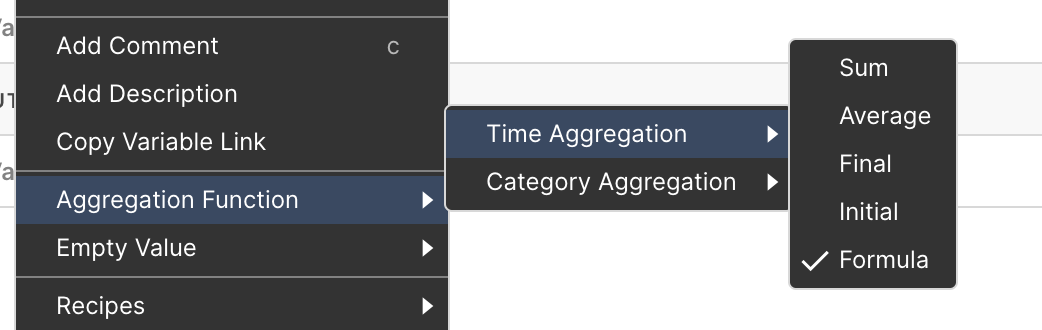#Causal updates causal.app

## Formula time aggregation

### New

The formula time aggregation uses the variable's formula to calculate an aggregation value. For example if you have a variable for the margin with the formula profit / revenue, the formula aggregation will take the total profit and divide it by the total revenue of the aggregated period.

You can change the aggregation function by right-clicking on the variable: